# colormap

View and set current colormap

## Syntax

``colormap map``
``colormap(map)``
``colormap(target,map)``
``cmap = colormap``
``cmap = colormap(target)``

## Description

example

````colormap map` sets the colormap for the current figure to one of the predefined colormaps. If you set the colormap for the figure, then axes and charts in the figure use the same colormap. The new colormap is the same length (number of colors) as the current colormap. When you use this syntax, you cannot specify a custom length for the colormap. To learn more about colormaps, see What Is a Colormap?```

example

````colormap(map)` sets the colormap for the current figure to the colormap specified by `map`.```

example

````colormap(target,map)` sets the colormap for the figure, axes, or chart specified by `target`, instead of for the current figure.```

example

````cmap = colormap` returns the colormap for the current figure as a three-column matrix of RGB triplets.```

example

````cmap = colormap(target)` returns the colormap for the figure, axes, or chart specified by `target`.```

## Examples

collapse all

Create a surface plot and set the colormap to `winter`.

```surf(peaks) colormap winter```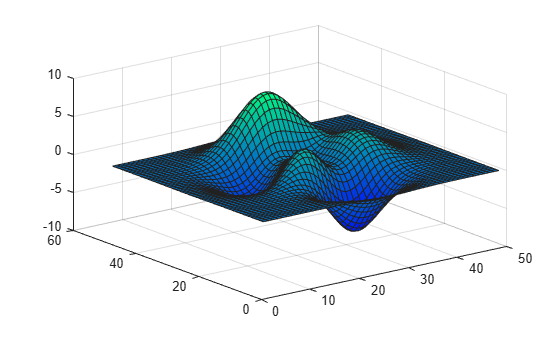First, change the colormap for the current figure to `summer`.

```surf(peaks) colormap summer```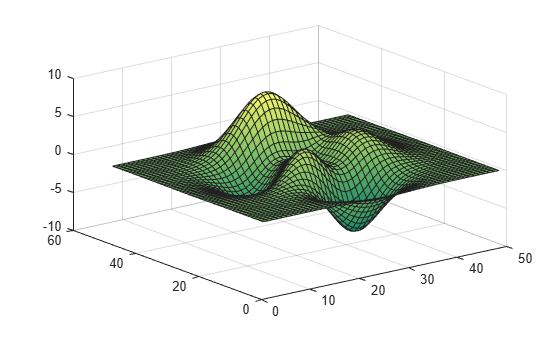Now set the colormap back to your system's default value. If you have not specified a different default value, then the default colormap is `parula`.

`colormap default`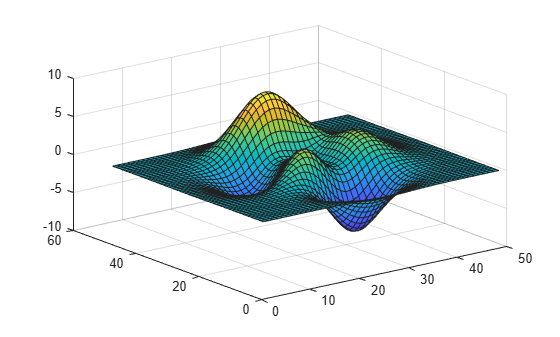Starting in R2019b, you can display a tiling of plots using the `tiledlayout` and `nexttile` functions. Call the `tiledlayout` function to create a 2-by-1 tiled chart layout. Call the `nexttile` function to create the axes objects `ax1` and `ax2`. Specify a different colormap for each axes by passing the axes object to the `colormap` function. In the upper axes, create a surface plot using the `spring` colormap. In the lower axes, create a surface plot using the `winter` colormap.

```tiledlayout(2,1) ax1 = nexttile; surf(peaks) colormap(ax1,spring) ax2 = nexttile; surf(peaks) colormap(ax2,winter)```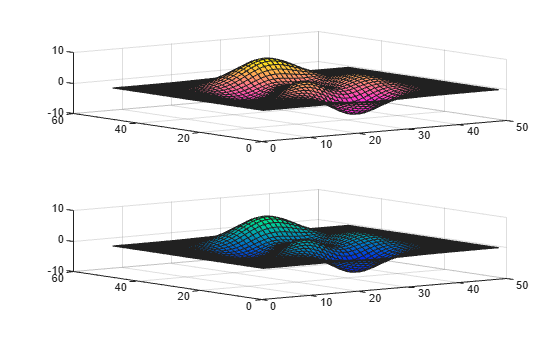Specify the number of colors used in a colormap by passing an integer as an input argument to the built-in colormap. Use five colors from the parula colormap.

```mesh(peaks) colormap(parula(5))```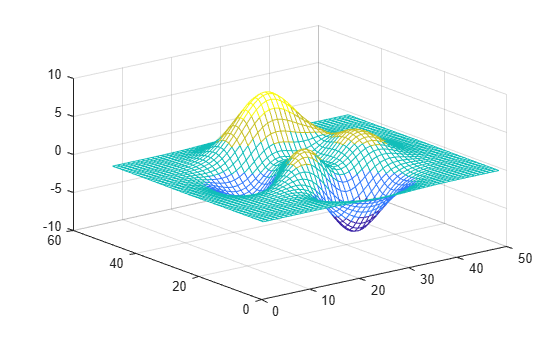Create a custom colormap by defining a three-column matrix of values between 0.0 and 1.0. Each row defines a three-element RGB triplet. The first column specifies the red intensities. The second column specifies the green intensities. The third column specifies the blue intensities.

Use a colormap of blue values by setting the first two columns to zeros.

```map = [0 0 0.3 0 0 0.4 0 0 0.5 0 0 0.6 0 0 0.8 0 0 1.0]; surf(peaks) colormap(map)```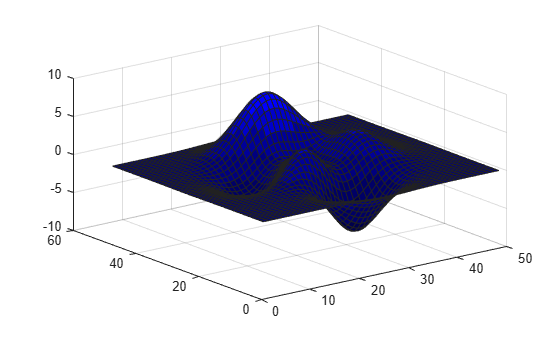Create a surface plot of the `peaks` function and specify a colormap.

```mesh(peaks) colormap(autumn(5))```Return the three-column matrix of values that define the colors used in the plot. Each row is an RGB triplet color value that specifies one color of the colormap.

`cmap = colormap`
```cmap = 5×3 1.0000 0 0 1.0000 0.2500 0 1.0000 0.5000 0 1.0000 0.7500 0 1.0000 1.0000 0 ```

Return the colormap values for a specific axes by passing the axes object to the `colormap` function.

Create a tiling of two plots using the `tiledlayout` and `nexttile` functions, which are new functions starting in R2019b. Call the `tiledlayout` function to create a 2-by-1 tiled chart layout. Call the `nexttile` function to create the axes objects `ax1` and `ax2`. Then display two filled contour plots with different colormaps.

```tiledlayout(2,1) ax1 = nexttile; contourf(peaks) colormap(ax1,hot(8)) ax2 = nexttile; contourf(peaks) colormap(ax2,pink)```Return the colormap values used in the upper plot by passing `ax1` to the `colormap` function. Each row is an RGB triplet color value that specifies one color of the colormap.

`cmap = colormap(ax1)`
```cmap = 8×3 0.3333 0 0 0.6667 0 0 1.0000 0 0 1.0000 0.3333 0 1.0000 0.6667 0 1.0000 1.0000 0 1.0000 1.0000 0.5000 1.0000 1.0000 1.0000 ```

Load the `spine` data set that returns the image `X` and its associated colormap `map`. Display `X` using the `image` function and set the colormap to `map`.

```load spine image(X) colormap(map)```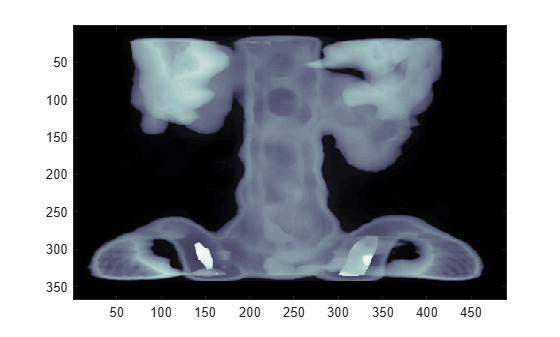## Input Arguments

collapse all

Colormap for the new color scheme, specified as a colormap name, a three-column matrix of RGB triplets, or `'default'`. A colormap name specifies a predefined colormap with the same number of colors as the current colormap. A three-column matrix of RGB triplets specifies a custom colormap. You can create the matrix yourself, or you can call one of the predefined colormap functions to create the matrix. For example, `colormap(parula(10))` sets the colormap of the current figure to a selection of 10 colors from the `parula` colormap.

A value of `'default'` sets the colormap to the default colormap for the target object.

#### Colormap Name

The following table lists the predefined colormaps.

Colormap NameColor Scale
`parula`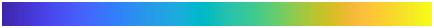`turbo``hsv`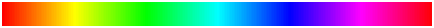`hot`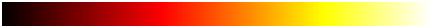`cool`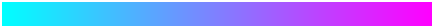`spring`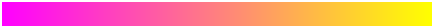`summer``autumn`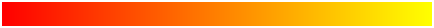`winter`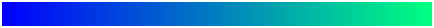`gray`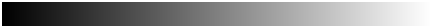`bone`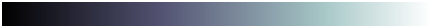`copper`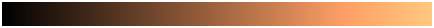`pink`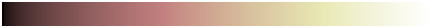`jet`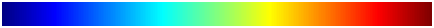`lines`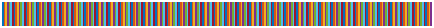`colorcube`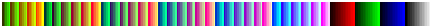`prism`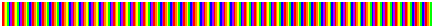`flag`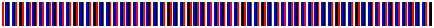`white`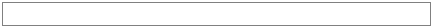#### Three-Column Matrix

To create a custom colormap, specify `map` as a three-column matrix of RGB triplets where each row defines one color. An RGB triplet is a three-element row vector whose elements specify the intensities of the red, green, and blue components of the color. The intensities can be `double` or `single` values in the range [0, 1], or they can be `uint8` values in the range [0, 255]. For example, this matrix defines a colormap containing five colors.

```map = [0.2 0.1 0.5 0.1 0.5 0.8 0.2 0.7 0.6 0.8 0.7 0.3 0.9 1 0];```

This table lists the RGB triplet values for common colors.

Color`double` or `single` RGB Triplet`uint8` RGB Triplet
yellow`[1 1 0]``[255 255 0]`
magenta`[1 0 1]``[255 0 255]`
cyan`[0 1 1]``[0 255 255]`
red`[1 0 0]``[255 0 0]`
green`[0 1 0]``[0 255 0]`
blue`[0 0 1]``[0 0 255]`
white`[1 1 1]``[255 255 255]`
black`[0 0 0]``[0 0 0]`

Data Types: `char` | `double` | `single` | `uint8`

Target, specified as one of these values:

• `Figure` object. The figure colormap affects plots for all axes within the figure.

• `Axes` object, `PolarAxes` object, or `GeographicAxes` object. You can define a unique colormap for the different axes within a figure.

• Graphics object that has a `Colormap` property. For example, you can change or query the colormap for a `HeatmapChart` object.

## Output Arguments

collapse all

Colormap values, returned as a three-column matrix of RGB triplets. Each row of the matrix defines one RGB triplet that specifies one color of the colormap. The values are in the range [0, 1].

collapse all

### What Is a Colormap?

A colormap is a matrix of values that define the colors for graphics objects such as surface, image, and patch objects. MATLAB® draws the objects by mapping data values to colors in the colormap.

Colormaps can be any length, but must be three columns wide. Each row in the matrix defines one color using an RGB triplet. An RGB triplet is a three-element row vector whose elements specify the intensities of the red, green, and blue components of the color. Typically, the intensities are `double` or `single` values in the range [0, 1]. A value of `0` indicates no color and a value of `1` indicates full intensity. For example, this command creates a colormap that has five colors: black, red, green, blue, and white.

```mymap = [0 0 0 1 0 0 0 1 0 0 0 1 1 1 1];```

To change the color scheme of a visualization, call the `colormap` function to change the colormap of the containing axes or figure. For example, the following commands create a surface plot and set the colormap of the figure to `mymap`.

```surf(peaks) colormap(mymap)```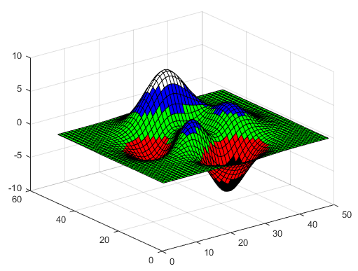## Tips

• To control the limits of the colormap, and how those limits relate to the range of your data, use the `caxis` function.

## Version History

Introduced before R2006a

expand all

Behavior changed in R2020b

Behavior changed in R2018a

Behavior changed in R2014b